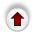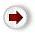# 1. Introduction To Trees

We will now turn to tree structures, which is the subject of most of the rest of the course.

### Definition of Trees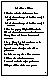Trees are as common and important as lists. And like lists there are many variations - binary search trees, balanced trees, and heaps are the main ones we will look at.

Recall that a list is a collection of components in which

1. each component (except one, the first) has exactly 1 predecessor.
2. each component (except one, the last) has exactly 1 successor.
a tree is very similar: it has property (1) but (2) is slightly relaxed:

(2') each component has some number of successors.

If there is no limit on the number of successors that a node can have, the tree is called a general tree.

If there is an maximum number N of successors for a node, then the tree is called an N-ary tree. In particular a binary (2-ary) tree is a tree in which each node has either 0, 1, or 2 successors.

In the lectures we will always use binary trees. It turns out this is not a restriction at all because all trees, even general trees, can be represented using binary trees (see text, (section 7.3.3)).

The unique node with no predecessor is called the root of the tree. A node with no successors is called a leaf - there will usually be many leaves in a tree. The successors of a node are called its children; the unique predecessor of a node is called its parent. If two nodes have the same parent, they are called brothers or siblings. In a binary tree the two children are called the left and right.

### Drawing Trees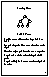Here is how we draw a tree:

The root is at the top; below it are its children. An arc connects a node to each of its children: we sometimes draw arrowheads on the arc, but they are optional because the direction parent->child is always top->bottom.

Then we continue in the same manner, the children of each node are drawn below the node.

For example, here is a binary tree:
`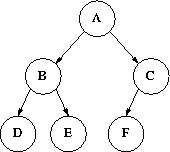`
In general, each child of a node is the root of a tree ``within the big tree''. For example, B is the root of a little tree (B,D,E), so is C. These inner trees are called subtrees. The subtrees of a node are the trees whose roots are the children of the node. e.g. the subtrees of A are the subtrees whose roots are B and C. In a binary tree we refer to the left subtree and the right subtree.

### Path in a Tree

A path is any linear subset of a tree, e.g. A-B-E and C-F are paths. The length of a path could be counted as either the number of nodes or the number of edges on the path - in the lectures we will count the nodes; e.g. A-B-E has length 3. But be careful: there is no agreed definition! The textbook defines path length as the number of edges.

There is a unique path from the root to any node. Simple as this property seems, it is extremely important: all our algorithms for processing trees will depend upon it. The depth or level of a node is the length of this path. When you draw a tree, it is very useful if all the nodes in the same level are drawn as a neat horizontal row. The depth or height of a tree is the maximum depth of the nodes in the tree.

### Ordered Trees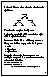A tree is ordered if there is some significance to the order of the subtrees. For example, consider this tree:

`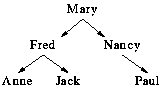`
If this is a family tree, there could be no significance to left and right. In this case the tree is unordered, and we could redraw the tree exchanging subtrees without affecting the meaning of the tree. On the other hand, there may be some significance to left and right - maybe the left child is younger than the right... or (as is the case here) maybe the left child has the name that is earlier in the alphabet. Then, the tree is ordered and we are not free to move around the subtrees.

For now we will restrict ourselves to ordered trees. Like lists, ordered N-ary trees have a nice recursive structural definition:

### Structural Definition of Binary Trees

A binary tree is either empty or it has 3 parts:

• a value
• a left subtree
• a right subtree
Whenever a data structure has a recursive definition like this, most of the `properties' of the data structure can be computed in a recursive manner which exactly mirrors the definition.

For example, here is a function to compute the number of nodes in a binary tree:

```        int size(binary_tree *t)
{
return is_empty(t) ? 0 : 1 + size(t->left) + size(t->right);
}```
With lists we had an alternative to recursion - we could scan through a list as easily with normal loops (`while`, `do ... while`) as with recursion. This is not true for trees. It is possible to scan through a tree non-recursively, but it is not nearly as easy as scanning recursively.